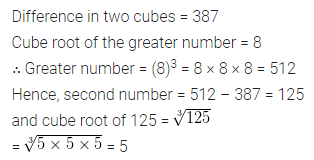## ML Aggarwal Class 8 Solutions for ICSE Maths Chapter 4 Cubes and Cube Roots Ex 4.2

Question 1.
Find the cube root of each of the following numbers by prime factorisation:
(i) 12167
(ii) 35937
(iii) 42875
(iv) 21952
(v) 373248
(vi) 32768
(vii) 262144
(viii) 157464
Solution: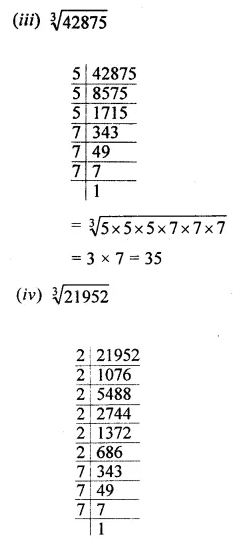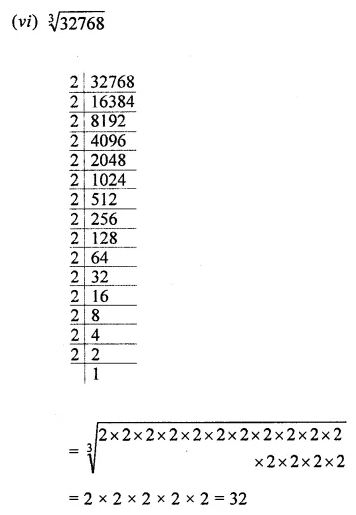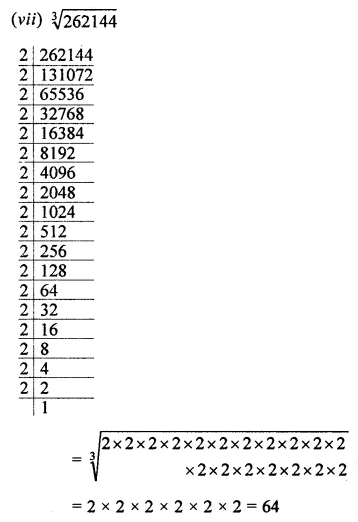Question 2.
Find the cube root of each of the following cube numbers through estimation.
(i) 19683
(ii) 59319
(iii) 85184
(iv) 148877
Solution: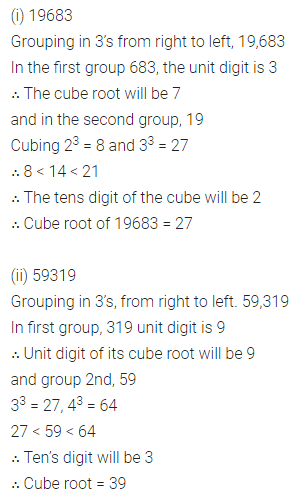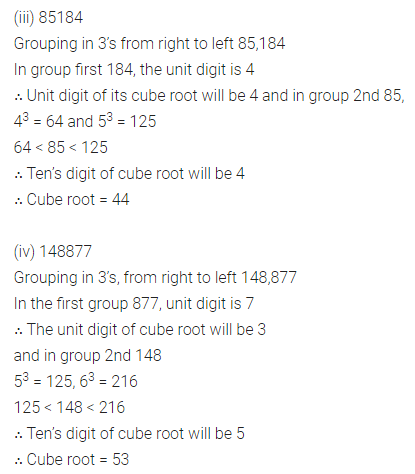Question 3.
Find the cube root of each of the following numbers:Solution: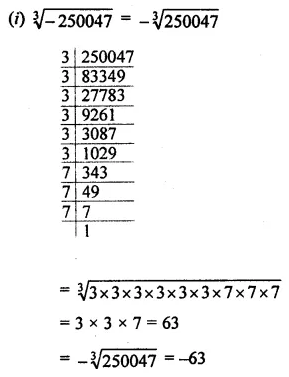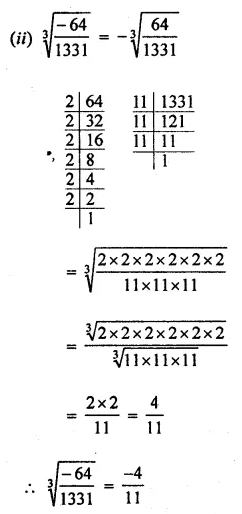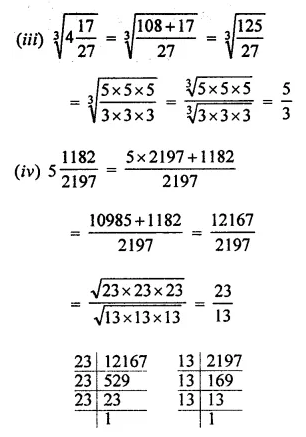Question 4.
Evaluate the following:
(i) $$\sqrt{512 \times 729}$$
(ii) $$\sqrt{(-1331) \times(3375)}$$
Solution:Question 5.
Find the cube root of the following decimal numbers:
(i) 0.003375
(ii) 19.683
Solution: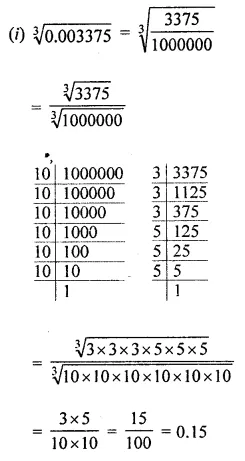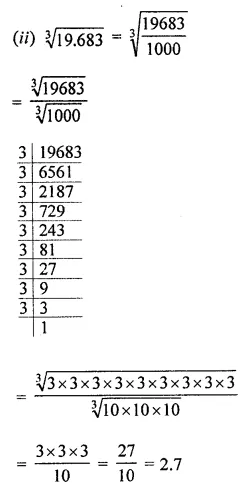Question 6.
Evaluate: $$\sqrt{27}+\sqrt{0.008}+\sqrt{0.064}$$
Solution: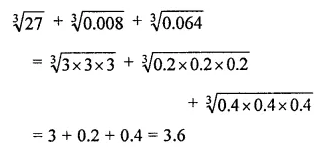Question 7.
Multiply 6561 by the smallest number so that product is a perfect cube. Also find the cube root of the product.
Solution: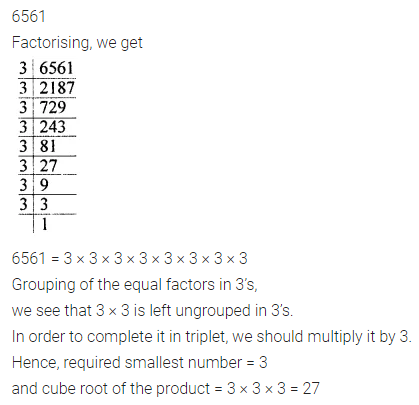Question 8.
Divide the number 8748 by the smallest number so that the quotient is a perfect cube. Also, find the cube root of the quotient.
Solution: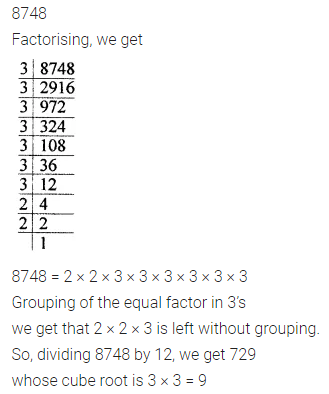Question 9.
The volume of a cubical box is 21952 m3. Find the length of the side of the box.
Solution: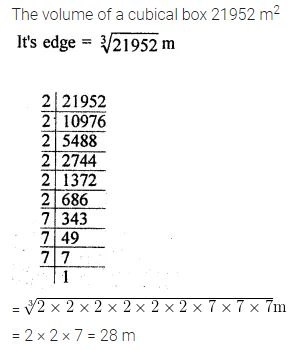Question 10.
Three numbers are in the ratio 3 : 4 : 5. If their product is 480, find the numbers.
Solution:Question 11.
Two numbers are in the ratio 4 : 5. If the difference of their cubes is 61, find the numbers.
Solution:Question 12.
The difference of two perfect cubes is 387. If the cube root of the greater of two numbers is 8, find the cube root of the smaller number.
Solution: